# Introduction, Functions Notes | Study Algebra for IIT JAM Mathematics - Mathematics

## Mathematics: Introduction, Functions Notes | Study Algebra for IIT JAM Mathematics - Mathematics

The document Introduction, Functions Notes | Study Algebra for IIT JAM Mathematics - Mathematics is a part of the Mathematics Course Algebra for IIT JAM Mathematics.
All you need of Mathematics at this link: Mathematics

Have you ever thought that a particular person has particular jobs or functions to do? Consider the functions or roles of postmen. They deliver letters, postcards, telegrams and invites etc. What do firemen do? They are responsible for responding to fire accidents. In mathematics also, we can define functions. They are responsible for assigning every single object of one set to that of another.

Functions
A function is a relation that maps each element x of a set A with one and only one element y of another set B. In other words, it is a relation between a set of inputs and a set of outputs in which each input is related with a unique output. A function is a rule that relates an input to exactly one output.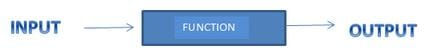It is a special type of relation. A relation f from a set A to a set B is said to be a function if every element of set A has one and only one image in set B and no two distinct elements of B have the same mapped first element.  A and B are the non-empty sets. The whole set A is the domain and the whole set B is codomain.

Representation
A function f: X →Y is represented as f(x) = y, where, (x, y) ∈ f and x ∈ X and y ∈ Y.
For any function f, the notation f(x) is read as “f of x” and represents the value of y when x is replaced by the number or expression inside the parenthesis. The element y is the image of x under f and x is the pre-image of y under f.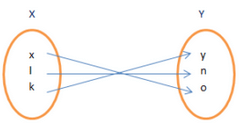Every element of the set has an image which is unique and distinct. If we notice around, we can find many examples of functions.
If we lift our hand upward, it is a function. Waving our hand freely, it is a function.  A walk in a circular track, yes it is a type of function. Now you can think of other examples too! A graph can represent a function. The graph is the set of all pairs of the Cartesian product.
Does this mean that every curve in the world defines a function? No, not every curve drawn is a function. How to find it? Vertical line test. If any curve intercepts a vertical line at more than one point, it is a curve only not a function.

Solved Example for You

Problem: Which of the following is a function?

1.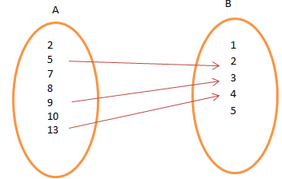2.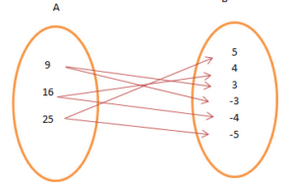3.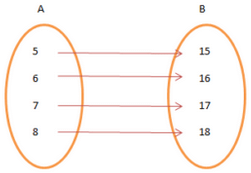Solution: Figure 3 is an example of function since every element of A is mapped to a unique element of B and no two distinct elements of B have the same pre-image in A.

The document Introduction, Functions Notes | Study Algebra for IIT JAM Mathematics - Mathematics is a part of the Mathematics Course Algebra for IIT JAM Mathematics.
All you need of Mathematics at this link: MathematicsUse Code STAYHOME200 and get INR 200 additional OFF

## Algebra for IIT JAM Mathematics

182 videos|58 docs

Track your progress, build streaks, highlight & save important lessons and more!

,

,

,

,

,

,

,

,

,

,

,

,

,

,

,

,

,

,

,

,

,

,

,

,

;